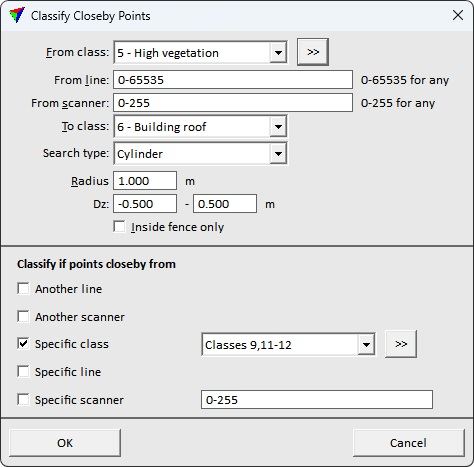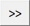# TerraScan User Guide

 Navigation: Batch Processing Reference > Classification Routines > Points Closeby points

Closeby points

Not Lite

Closeby points routine classifies points close to points from another class, another line, or another scanner. For each point, the software finds points within a given 2D or 3D search radius. It checks whether the points within the radius fulfill the given conditions, such as being in a specific class, from another line or scanner. If all defined conditions are true, the point is classified.

Example of a high-density point cloud:

thin the point cloud, keep a central point and classify other points into a temporary point class

apply classification routines to the thinned point cloud, classify points into any object-specific point classes

apply the Closeby points routine in order to densify the object classes with points from the temporary point class

In the above example, the processing speed is increased by thinning a dense point cloud before running processing-intensive classification routines. The Closeby points routine is fast to apply for a large number of points.

Example for a multiple-scanner system data set:

scanner 1 is better quality than scanner 2

classify ground from each scanner into separate classes

use ground points of scanner 2 only in places where there are no ground points of scanner 1.SETTING

EFFECT

From class

Source class(es).Opens the Select classes dialog which contains the list of active classes in TerraScan. You can select multiple source classes from the list that are then used in the From class field.

From line

Only points in the given line(s) are effected. Use a comma or minus to separate several line numbers, for example 2-5,10. 0-65535 refers to all lines.

From scanner

Only points captured by the given scanner(s) are effected. Use a comma or minus to separate several scanner numbers, for example 1-3,5. 0-255 refers to all scanners.

To class

Target class. Chose Closest point class if you want to deduce the class of a point in the source class(es) from the closest point in the Specific class selection.

Search type

2D - a 2D search radius (horizontal dimension) is applied for finding closeby points.

2D above - points are only classified if the reference points are above and within a 2D search radius.

2D below - points are only classified if the reference points are below and within a 2D search radius.

3D - a 3D search radius is applied for finding closeby points.

3D above - points are only classified if the reference points are above and within a 3D search radius.

3D below - points are only classified if the reference points are below and within a 3D search radius.

Size of the search radius around each point.

Inside fence only

If on, only points inside a fence or selected polygon(s) are classified.

Another line

If on, a point is classified if another point from any other line is within the given search radius.

Another scanner

If on, a point is classified if another points from any other scanner is within the given search radius.

Specific class

If on, a point is classified if another point from the given class(es) is within the given search radius.Opens the Select classes dialog which contains the list of active classes in TerraScan. You can select multiple source classes from the list that are then used in the Specific class field.

Specific line

If on, a point is classified if another point from the given line(s) is within the search radius. Use a comma or minus to separate several line numbers, for example 2-5,10.

Specific scanner

If on, a point is classified if another point from the given scanner(s) is within the search radius. Use a comma or minus to separate several scanner numbers, for example 1-3,5.

TerraScan User Guide   26.07.2021   © 2021 Terrasolid Ltd